# Java Data Types

Programmers can represent various types of values and carry out various operations using Java's diverse data types. We'll look at these data types' functionality and how to use them in Java programs.

Java defines the following eight primitive data types:

1. byte
2. short
3. char
4. int
5. long
6. float
7. double
8. boolean

Simple types is another name for the primitive data types. These fall into the following four categories:

1. Integers: This category includes byte, short, int, and long, all of which are whole-valued signed numbers.
2. Floating-point numbers: This category includes float and double, which represent numbers with fractional precision.
3. Characters: This category includes char, which represents the symbols in a character set, such as letters and numbers.
4. Booleans: This category includes booleans, which are special types used to represent true or false values.

Now I'm going to describe these four categories of data types one by one, starting with "integers".

You can use these types as is or construct arrays or your own class types from them. As a result, they serve as the foundation for all other types of data that can be generated.

## Java Data Types: Integers

Java defines these four integer types:

1. byte
2. short
3. int
4. long

These are all signed, positive and negative values. Unsigned, positive-only integers are not supported in Java. Many other programming languages support both signed and unsigned integers.

In any case, the designers of Java felt that unsigned integers were unnecessary. They believed that the concept of unsigned was primarily used to specify the behavior of a high-order bit, which defines the sign of an integer value. Java handles the significance of high-ordered bits in a unique way by including a special "unsigned right shift" operator. As a result, the requirement for an unsigned integer type was dropped.

The width of an integer type should be understood as the behavior it defines for the variables and expressions of that data type, rather than the amount of storage it consumes. The Java run-time environment, on the other hand, is always free to use whatever size it wants as long as the types behave as you declared them.

The widths and ranges of these integer data types vary widely, as shown here in this table:

Name Width Range
long 64 -9,223,372,036,854,775,808 to 9,223,372,036,854,775,807 -263 to 263 -1
int 32 -2,147,483,648 to 2,147,483,647 -231 to 231 -1
short 16 -32,768 to 32,767 -215 to 215 -1
byte 8 -128 to 127 -27 to 27 -1

### byte

The smallest integer type is a "byte." This is an 8-bit signed data type with a -128 to 127 range. Variables of type byte are particularly useful when working with data streams from a network or file. They are also useful when dealing with raw binary data that may not be directly compatible with other built-in Java types.

The "byte" keyword is used to declare byte variables. For example, the following code declares two byte variables, b and c:

`byte b, c;`

### short

"short" is a 64-bit signed type. It has a range of -32,768 to 32,767. It is the least common type. Here are some short variable declaration examples:

```short s;
short t;```

### int

The most common integer data type, as you may know, is "int." It is a 32-bit signed type with a range of -2,147,483,648 to 2,147,483,647.

In addition to other applications, int type variables are frequently used to control loops and index arrays. Even though you might think that using a byte or short is more efficient than using an int in situations where the larger range of an int data type isn't required, this isn't always the case. Because byte and short values are promoted to int when an expression is evaluated, they are used in expressions. When an integer is required, int is the best choice.

### long

"long" is a signed 64-bit type and is useful for those occasions where an int data type is not large enough to hold the desired value. The range of a long is quite large. This makes it helpful when big, whole numbers are needed. For instance, here is a program that computes the number of miles that light will travel in a given number of days:

```public class JavaProgram
{
public static void main(String args[])
{

int lightspeed;
long days;
long seconds;
long distance;

// approximate speed of light in miles per second
lightspeed = 186000;

days = 1000;    // specifies the number of days here

seconds = days * 24 * 60 * 60;    // convert to seconds

distance = lightspeed * seconds;     // compute distance

System.out.print("In " +days);
System.out.print(" days light will travel about " + distance + " miles.");
}
}
```

When the preceding Java program is compiled and run, the following output is produced: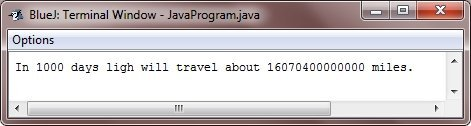Obviously, the outcome could not have been stored in an int variable.

## Java Data Types: Floating-point numbers

Floating-point or real numbers are used when evaluating the expressions that require fractional precision. For instance, calculations like square root or transcendentals such as sine and cosine result in a value whose precision requires the floating-point type.

Java implements the standard (IEEE-754) set of floating-point types and the operators. There are two kinds of floating-point types, namely, float and double, which represent single-precision and double-precision numbers, respectively.

The following table shows the width and range of the floating-point types:

Name Width in bits Approximate Range
double 64 4.9e-324 to 1.8e+308
float 32 1.4e-045 to 3.4e+038

### float

The type float specifies a single-precision value that uses 32 bits of storage. Single precision is faster on some processors and takes half as much space as double precision, but will become inaccurate when the values are either very large or very small.

Variables of the type float are useful when you need a fractional component but do not require a high degree of precision. For instance, float can be useful whenever representing dollars and cents.

Below are some examples of float variable declarations:

`float hightemp, lowtemp, rad;`

### double

Double precision is denoted by the keyword double, which uses 64 bits to store a value.

Double precision is actually faster than single precision on several modern processors that have been optimized for high-speed mathematical calculations. All transcendental math functions, like sin(), cos(), and sqrt(), return double values. When you need to maintain accuracy over many repetitive calculations or are manipulating large numbers, doubling is the best choice.

The following is a short and simple program that uses the double variables to compute the area of a circle:

```public class JavaProgram
{
public static void main(String args[])
{
double pi, r, a;

r = 10.8;          // radius of the circle
pi = 3.1416;       // approximate value of pi
a = pi * r * r;    // compute area of the circle

System.out.println("Area of Circle is " +a);
}
}```

When the above Java program is compiled and run, it will produce the following output: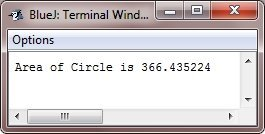## Java Data Types: Characters

The data type used to store characters in Java is called char. C/C++ programmers should be aware that Java's char is different from its counterpart in C/C++. char is 8 bits wide in C/C++. Java does not operate in this way. Unicode is used by Java to represent characters instead.

Unicode can represent all human language characters. It unites dozens of character sets, including Latin, Arabic, Greek, Hebrew, Cyrillic, and others. Unicode required 16 bits at Java's creation. Thus, Java char is 16-bit. Chars have a 0–65,536 range. No negative characters.

As usual, ASCII ranges from 0 to 127, and ISO-Latin-1 covers 0 to 255. Java uses Unicode to represent characters because it allows global programming. Unicode is inefficient for languages like English, Spanish, German, and French, whose characters can be controlled in 8 bits. But global portability costs this.

Below is a program that demonstrates the char variables:

```public class JavaProgram
{
public static void main(String args[])
{

char ch1, ch2;

ch1 = 88;        // ASCII code for 'X'
ch2 = 'Y';

System.out.print("ch1 : " + ch1 + "\nch2 : " + ch2);
}
}```

When the above Java program is compiled and run, it will produce the following output: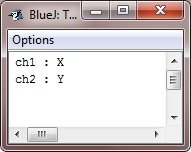Notice here that variable ch1 is assigned the value 88, which is the ASCII (and Unicode) value that corresponds to the letter X. As we've talked about, the first 127 values in the Unicode character set are used by the ASCII character set.For this reason, all the older tricks that you may have used with characters in other languages will work in Java, too.

Even though char is designed to hold the Unicode characters, it can also be used as an integer type on which you can perform arithmetic operations. For instance, you can add two characters together or increment the value of a character variable. Consider the following example program:

```public class JavaProgram
{
public static void main(String args[])
{

char ch1;

ch1 = 'X';
System.out.println("ch1 contains " +ch1);

ch1++;
System.out.println("ch1 is now " + ch1);
}
}```

When the above Java program is compiled and run, it will produce the following output: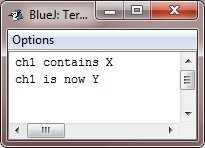In the above program, ch1 is first given the value X. Next, ch1 is incremented. This results in ch1 holding Y, the next character in the ASCII (and Unicode) sequence.

### Escape Sequences in Java

A character preceded by the backslash (\) is an escape sequence and has particular meaning to the compiler.

As you see, the newline character (\n) has been used frequently in System.out.println() statements to advance to the next line after the string is printed.

Look at the table given here, which shows Java's escape sequences:

Escape Sequence Description
\n Inserts a newline
\b Inserts a backspace
\f Inserts a form feed
\r Inserts a carriage return
\' Inserts a single quote character
\" Inserts a double quote character
\\ Inserts a backslash character
\t Inserts a tab

When an escape sequence is encountered in a print statement, the compiler interprets it accordingly. If you want to put quotes within quotes, then you must use the escape sequence, \", on the interior quotes. Let's look at the following example:

```class JavaProgram
{
public static void main(String args[])
{
System.out.println("He said \"Hello!\" to me.");
}
}```

It will produce the following result:## Java Data Types: Booleans

Java has a primitive type, called boolean, for the logical values. It can have only one of the two possible values, true or false. This is the type returned by all the relational operators, as in the case of a<b.

boolean is also the type needed by conditional expressions that govern control statements such as if and for.

Following is a program that illustrates the boolean type:

```public class JavaProgram
{
public static void main(String args[])
{
boolean b;

b = false;
System.out.println("b is " +b);
b = true;
System.out.println("b is " +b);

if(b)
System.out.println("This is executed.");

b = false;
if(b)
System.out.println("This is not executed.");

System.out.println("10 > 9 is " +(10>9));
}
}```

When the above Java program is compiled and executed, it will produce the following output: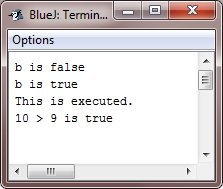There are three interesting things to notice about the above program. First, as you can see, when a boolean value is output by the method println(), "true" or "false" is displayed. Second, the value of a boolean variable is sufficient, by itself, to control the if statement. There is no requirement to write an if statement like this :
if(b == true) ...

Third, the outcome of a relational operator, such as <, is a boolean value. This is why the expression 10>9 displays the value "true". And the extra set of parentheses around 10>9 is necessary because the + operator has a higher precedence than >.

## Java Number

You are free to work with numbers in Java, using basic data types such as byte, int, double, long, etc. Here are some examples of defining and initializing numbers into variables in Java:

```int numi = 432;
float numf = 43.23;```

For example:

```class JavaProgram
{
public static void main(String args[])
{
Integer num = 50;
num =  num + 100;
System.out.println(num);
}
}```

Here is the output that the aforementioned Java program produced: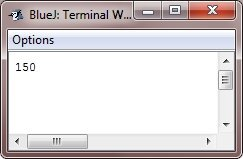Java Online Test

« Previous Tutorial Next Tutorial »